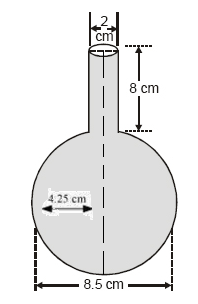# A spherical glass vessel has a cylindrical neck 8 cm long, 2 cm in diameter; the diameter of the spherical part is 8.5 cm. By measuring the amount of water it holds, a child finds its volume to be 345 cm3. Check whether she is correct, taking the above as the inside measurements, and π = 3.14.Given

For the cylinder part, Height (h) = 8 cm and Radius (R) = (2/2) cm = 1 cm

For the spherical part, Radius (r) = (8.5/2) = 4.25 cm

Find out

We have to verify whether the measurements taken by the child are accurate

Amount of water it can hold = Total Volume of this vessel

= Volume of cylinder + Volume of a sphere

= πr2h + 4/3πR3

= 8π + 102.35π

On considering π=3.14

= 110.35π

= 346.499 cm3

Volume measured by child = 345 cm3, which is not right

Correct volume = 346.5 cm3

Hence, the Correct volume = 346.5 cm3